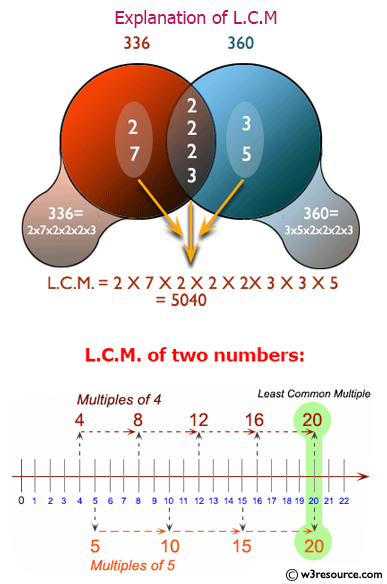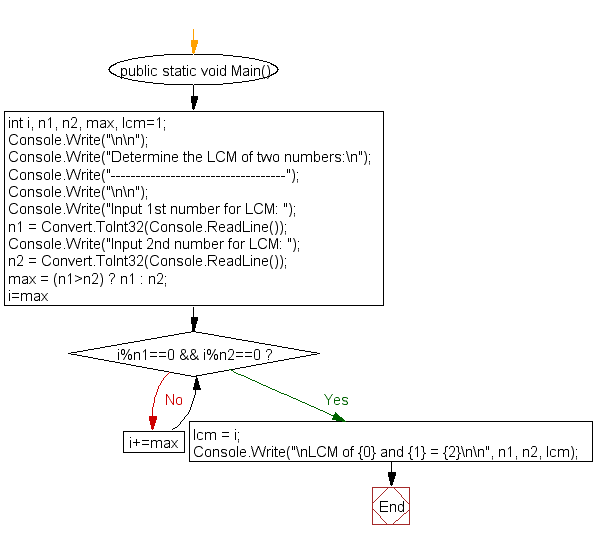﻿ C# - Determine the LCM of two numbers - w3resource# C# Sharp Exercises: Determine the LCM of two numbers

## C# Sharp For Loop: Exercise-45 with Solution

Write a program in C# Sharp to find LCM of any two numbers.

Pictorial Presentation:Sample Solution:

C# Sharp Code:

``````using System;
public class Exercise45
{
public static void Main()
{
int i, n1, n2, max, lcm=1;
Console.Write("\n\n");
Console.Write("Determine the LCM of two numbers:\n");
Console.Write("-----------------------------------");
Console.Write("\n\n");
Console.Write("Input 1st number for LCM: ");
Console.Write("Input 2nd number for LCM: ");
max = (n1>n2) ? n1 : n2;
for(i=max;  ; i+=max)
{
if(i%n1==0 && i%n2==0)
{
lcm = i;
break;
}
}
Console.Write("\nLCM of {0} and {1} = {2}\n\n", n1, n2, lcm);
}
}
```
```

Sample Output:

```Determine the LCM of two numbers:
-----------------------------------
Input 1st number for LCM: 6
Input 2nd number for LCM: 8
LCM of 6 and 8 = 24
```

Flowchart:C# Sharp Code Editor:

Contribute your code and comments through Disqus.

What is the difficulty level of this exercise?

Test your Programming skills with w3resource's quiz.

﻿